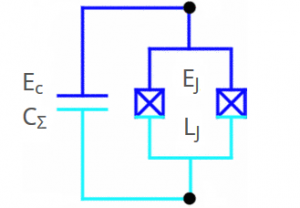# Anton Potočnik

at imec

# Transmon Qubit Calculator

With this calculator you can convert between charging energy (Ec) and total capacitance (CΣ), between Josephson energy (EJ), Josephson inductance (LJ), junction critical current (Ic) or room temperature junction resistance (R), and from charging and Josephson energies calculate relevant qubit transition frequencies. Other calculations are added below including Qubit’s Purcell decay, chi-shift, conversion between spectral linewidth and coherence times, etc.

All frequencies are in natural units.

#### Al Josephson Junction/SQUID

 Ec/2π = GHz CΣ = fF
 EJ/2π = GHz LJ = nH Ic = nA R = Ω

#### Qubit frequencies

 fge ≈ GHz fef ≈ GHz fgf/2 ≈ GHz α ≈ GHzSchematic of a transmon qubit.

#### Purcell decay and χ-shift

 fres = GHz Qres = k κres = MHz g/2π = MHz
 Δ = fge – fres = GHz TPurcell ≈ μs κPurcell ≈ kHz χ-shift ≈ MHz ncrit ≈

#### Coherence times / spectral linewidth

 T1 = μs T2 = μs Tφ = μs
 f = GHz dffwhm = kHz Q-factor = M

Tagged under: